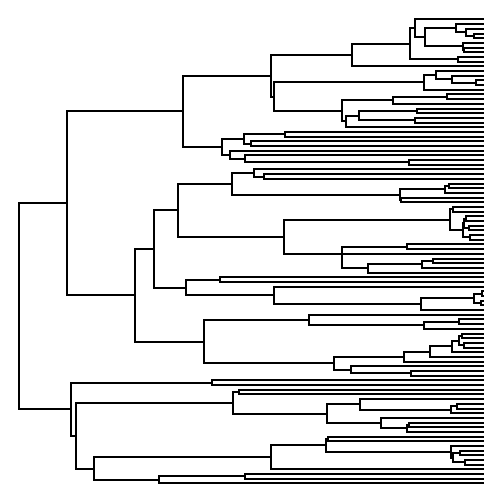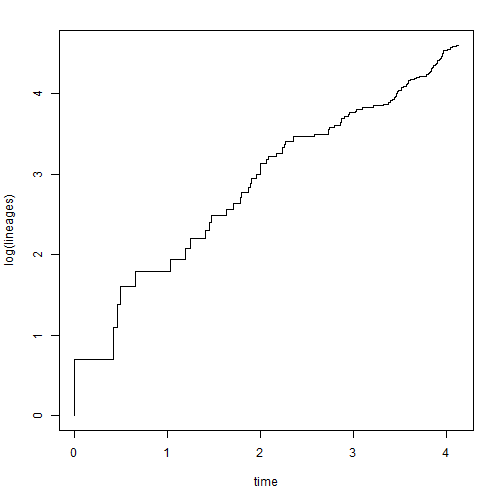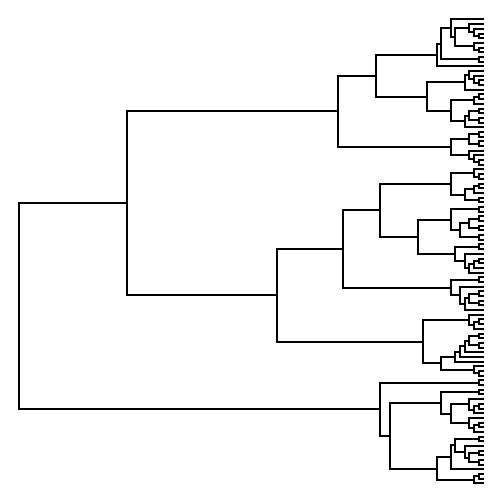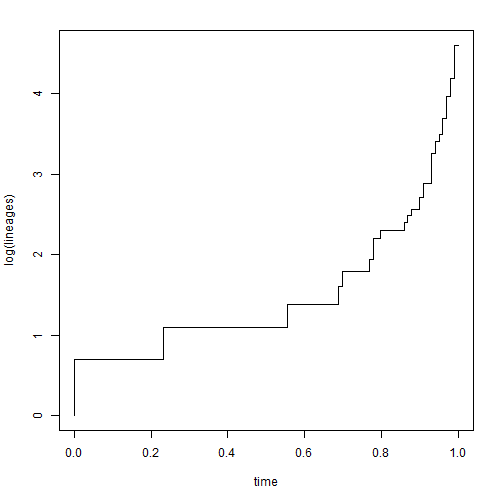## Monday, April 13, 2015

### Sampling edge lengths under a Yule process

There has been a little bit of discussion today on R-sig-phylo listserve about transforming branch lengths.

One thing that wasn't mentioned was the possibility of sampling branch lengths under a model. I thought it would be straightforward to sample branch lengths under a Yule model (that is, a pure-birth speciation model).

The following is code that does this. (Set `plot=TRUE` to see a cool animation of the tree being 'grown' from left to right.)

``````pb_edgelength<-function(tree,b=1,plot=TRUE,...){
ll<-rexp(n=Ntip(tree)-1,rate=2:Ntip(tree)*b)
tree\$edge.length<-rep(0,nrow(tree\$edge))
live.nodes<-Descendants(tree,Ntip(tree)+1,"children")
tips<-vector()
for(i in 1:length(ll)){
tips<-c(tips,live.nodes[live.nodes<=Ntip(tree)])
live.nodes<-setdiff(live.nodes,tips)
ii<-which(tree\$edge[,2]%in%c(live.nodes,tips))
tree\$edge.length[ii]<-tree\$edge.length[ii]+ll[i]
node<-if(length(live.nodes)<=1) live.nodes else
sample(live.nodes,1) ## choose one node
live.nodes<-c(setdiff(live.nodes,node),
Descendants(tree,node,"children"))
if(plot) plotTree(tree,...)
}
tree
}
``````

OK, now let's try it out with a tree obtained using `rtree` from the ape package:

``````library(phytools)
library(phangorn)
tree<-rtree(n=100,br=NULL) ## no branch lengths
t.pb<-pb_edgelength(tree,plot=FALSE)
plotTree(t.pb,ftype="off")
````````````par(mar=c(5.1,4.1,2.1,2.1))
obj<-ltt(t.pb)
````````````obj\$gamma
``````
``````##  -0.1807099
``````

Compare this to Grafen's edge lengths from `compute.brlen`:

``````t.grafen<-compute.brlen(tree)
plotTree(t.grafen,ftype="off")
````````````par(mar=c(5.1,4.1,2.1,2.1))
obj<-ltt(t.grafen)
````````````obj\$gamma
``````
``````##  6.732706
``````

Obviously, the branching times from Grafen's branch length transformation are very different from those obtained under a Yule process!

That's it, really.